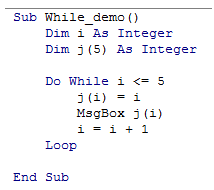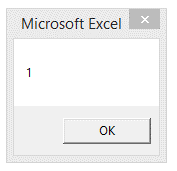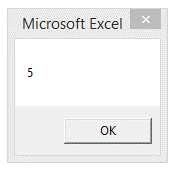## VBA Do While loop in Excel

Do While loop is similar to For loop except that the increment is defined within the loop.

The script within the loop executes as long as the condition specified in the Do While statement is true.

This loop always end with "Loop" keyword.

Syntax

1. Do While
2. /code for what should be done here
3. Loop

Example: The following code executes as long as Integer variable "I" is less than 5.

1. Sub While_demo()
2. Dim i As Integer
3. Dim j(5) As Integer
4. Do While i <= 5
5. j(i) = i
6. MsgBox j(i)
7. i = i + 1
8. Loop
9. End Sub

A glance at the editorial screenshot is shown below.Result: